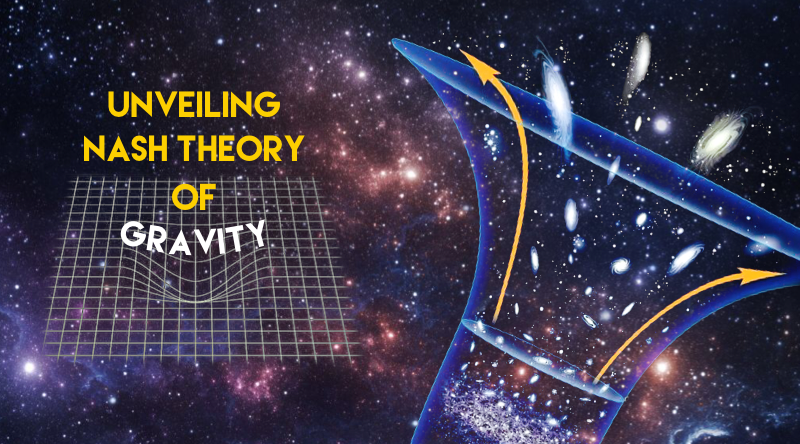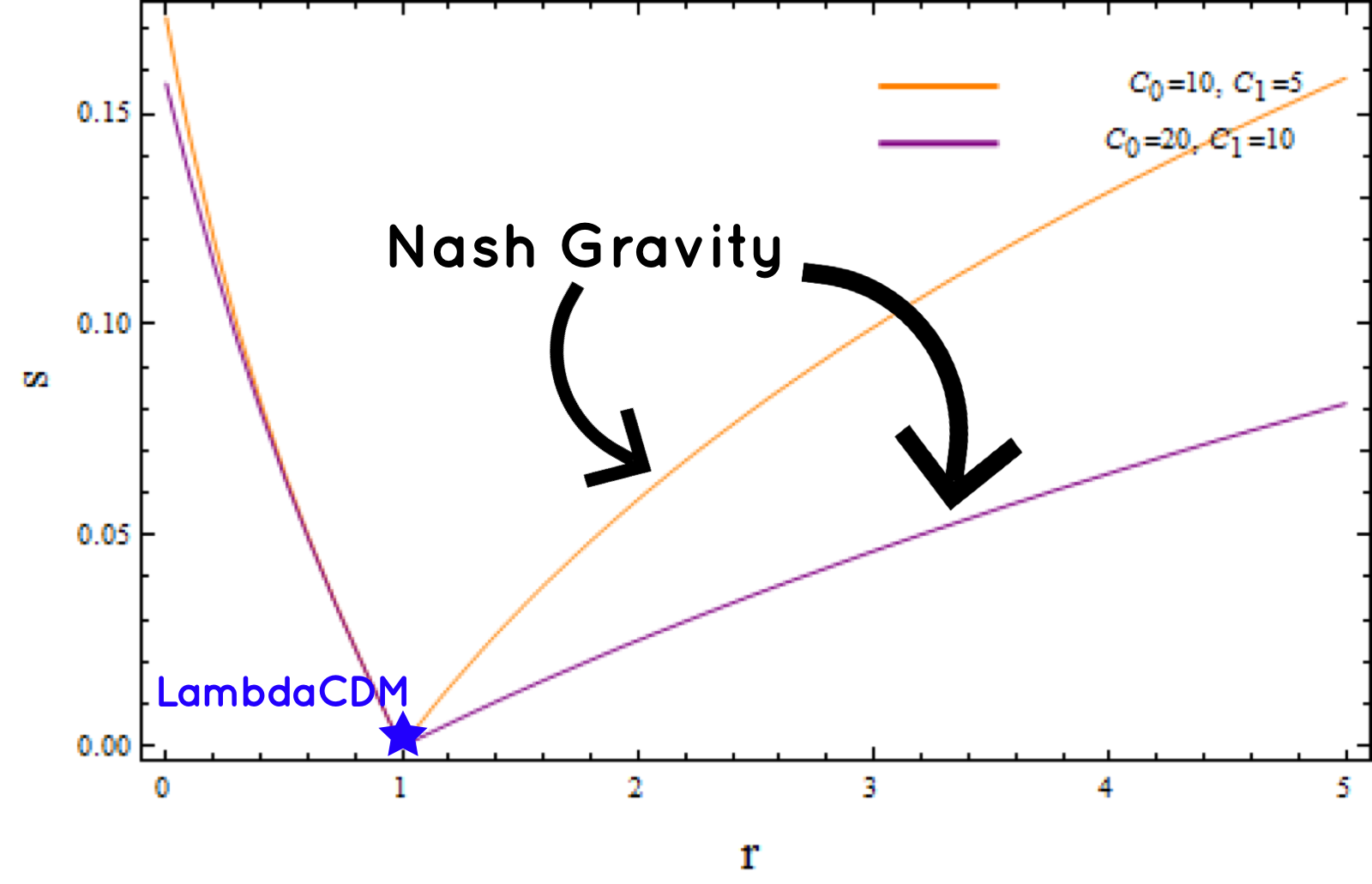# Unveiling Nash theory of gravity

the cosmological implications of Nash theory of gravity

When Einstein developed his famous theory of gravity, he suggested that the universe was finite and static neither expanding nor collapsing. Later in the late 1990s, teams of researchers discovered that the universe is in the phase of an accelerating expansion. However, the standard model of cosmology using Einstein theory of gravity cannot explain this behavior. Physicists have been trying to figure out what drives this cosmic expansion. Regarding the late-time cosmic acceleration, there are at least two promising explanations, to date. One of them is to introduce “dark energy” in the context of Einstein theory. However, the dark energy remains still unknown.Another attractive approach is to consider the modification of Einstein gravity on the large-scale methodology. An interesting alternative theory of gravity was proposed by a renowned mathematician John Nash in 1900s. The theory was intended just to replace Einstein’s gravity theory. Nash simply wanted to see how the gravity is altered by the presence of energy. However, Assoc. Prof. Dr. Phongphichit Channuie at Walailak University in collaboration with Dr.Davood Momeni & Dr.Mudhahir Al Ajmi — researchers at Sultan Qaboos University in Oman, has considered its cosmological implication. In this research, equations of motion were formulated based on homogeneous and anisotropic space-time (Bainchi-type I model). The exact solutions of the proposed equation of motion were derived using Noether symmetry technique. Prominently, the solutions could explain the accelerating expansion of the universe at late time. This work demonstrates the very first implications of Nash theory of gravity in cosmology.

Sources: